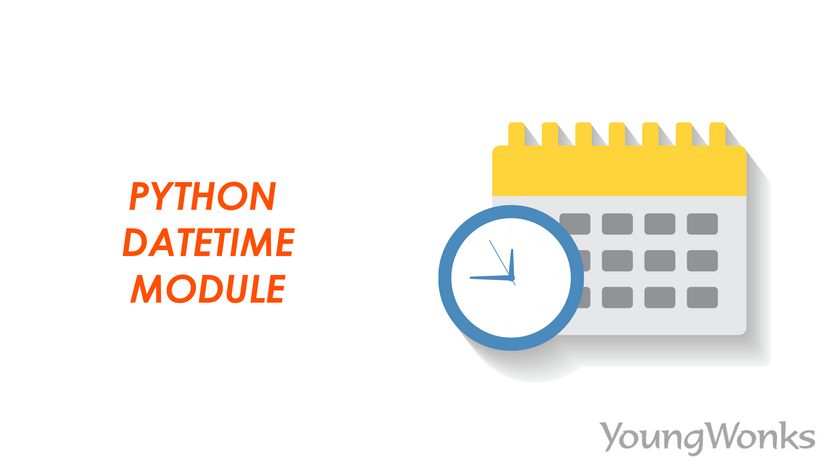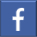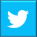# Python-Datetime-Module

## This blog gives in-depth knowledge of the various functions available in the Python datetime module, how is it different from the time module and how these functions can be used. Read our blog to learn more...Feb 19, 2023    By Team YoungWonks *

## Introduction

Python is thought to be one of the most basic programming languages. Because of the built-in modules, this programming language is simple to learn and use. Users can create applications and algorithms much faster with the help of built-in libraries than with other programming languages. It includes simple libraries such as random, time, datetime, and calendar, as well as libraries such as flask, Django, numpy, and pandas, which aid in web development and data analytics.

In this blog tutorial, we will go over the functionality of one such module, the datetime module. We will go over the functions and how they are used in detail.

## What is Python datetime module and why is it used?

Python includes a built-in module called datetime. Because Python does not have time and date as distinct data types, it is used to manipulate and represent them. The Datetime module contains various functions for formatting date and time, such as determining the current time or date based on inputs, time zone names, time zone information, and so on. This blog will go over all of these functions.

Because dates and times are not data types in Python. This module can be used directly, making it easier for developers to work.

## How is datetime module different from the time module in Python?

The Time module only deals with time attributes such as seconds, microseconds, hours, minutes, and tzone. This module lacks date attributes and thus cannot be used to represent dates. The datetime module, on the other hand, can be used to represent both date and time. At the same time, it can include attributes such as month, day, year, hour, second, and microsecond. This is the primary distinction between the modules.

## How to use the Python datetime module?

Since the Python datetime module is built-in, it is included with the Python package. We only need to import the time module in order to use it.

Syntax: import datetime

## Types of classes in the datetime module

This module contains six different types of classes (constructors). Each class has a number of class methods (functions) that can be used. These classes are used to create datetime objects.

1. Date:

The year, month, and date are the three attributes of the date class. It also only accepts these three attributes as arguments. The numeric date is converted into a date object here.

2. Time:

The Time class is used to display time data regardless of the date or day. Hour, minute, second, microsecond, and tzinfo are the attributes.

3. Datetime:

This class is used to determine the date and time combination. This includes the following attributes: year, month, date, hours, minutes, seconds, microseconds, and tzinfo.

4. Timedelta:

The Timedelta class is used to show the difference in date or time at any given point. It shows up in microseconds.

5. Tzinfo:

We can obtain time zone information by using tzinfo.

6. Timezone:

The datetime module's final class provides time zone information from UTC. We will be using the most recent 3.2 version of UTC. This is highly used in web development.

## Functions in the datetime module

The various classes have already been discussed in the datetime module introduction. Let us now examine the functions of each class.

### 1. Current Date

The date class is used to retrieve the current local date from the date object. The Gregorian calendar is always used by the date class.

Syntax:

datetime.date.today()

or

from datetime import date date.today()

The output in the following example returns the current date. There are two ways to use the function. One method is to use the datetime module directly, while the other is to use the module's date class. The attributes of a date object can also be extracted. Take a look at the following example.

### 2. Create a date object

Using the date function, we can create a date object. This function requires three parameters: year, month, and date. It returns the date in the format yyyy-mm-dd.

Each argument has a set of criteria that must be met, as listed below:

Year: Minimum year(maxyear) <= Year <= Maximum year

Month: 1 <= Month <=12

Date: 1 <= Date <= Maximum number of days in a given month.

Syntax:

datetime.date(year, month, date)

The arguments in this function must be kept in the same order or Python will throw a value error.

### 3. Date from timestamp

We can get the date from the timestamp method. The number of seconds are calculated between any given date and January 1, 1970, at UTC.

Syntax:

date.fromtimestamp()

### 4. Create a time object

To create a time object, we can use the time class. It requires 5 arguments in the same order: hour, minute, second, microsecond, and fold. This function accepts optional arguments with the default value of 0. The output is in the format hh:mm:ss. If we do not provide an argument, it will return 00:00:00.

Syntax:

datetime.time (hours, minutes, seconds, microseconds)

or

from datetime import time

time(hours, minutes, seconds, microseconds)

### 5. Current time function

The return value of this function is the current year, date and time along with the day. This also takes the number of seconds since epoch as the argument.

Syntax:

time.ctime (n)

Until now we have seen how we get the struct_time object. Now let us learn how to do the other way around. We will make use of a time tuple which is a named tuple. Note: If no argument is present it calculates the time till present.

### 6. Current date and time

To determine the current date and time, we use the datetime class. The user can use this function to determine the local time and date. It returns the output in YYYY-MM-DD hh:mm:ss:ms format.

Syntax:

from datetime import datetime

datetime.now()

### 7. Create a datetime object

The arguments year, month, date, hours, minutes, seconds, and milliseconds are required. The arguments are formatted as YYYY-MM-DD hh:mm:ss:ms. As a result, a datetime object is returned.

Syntax:

from datetime import datetime

datetime(year, month, day, hour, minute, second)

### 8. Difference between time and date

The class datetime.timedelta is used to calculate the difference between two dates or times.

As arguments, it accepts weeks, days, hours, minutes, seconds, milliseconds and microseconds. These arguments, however, are optional. To get the output, we can add or subtract the two timedelta objects.

Syntax:

from datetime import timedelta

td1 = timedelta(weeks, days, hours, seconds)

td2 = timedelta(days, hours, minutes, seconds)

result = td2-td1

### 9. Tzinfo Class

The functions listed above do not provide the ability to obtain time zone information. However, time zone is an important consideration because different locations on the globe have different time zones. Therefore we make use of the tzinfo which is an abstract class. This class cannot be accessed directly. It's called the naive datetime object and has the value none.

datetime.tzinfo = None

We use the pytz module to solve this problem. To use it, we directly import this built-in module.

Syntax:

import pytz

The UTC time is obtained using this module. It also takes into account daylight savings time automatically (dst). It provides information by indicating whether dst is 0 or 1.

Syntax:

pytz.timezone(location)

In the datetime function, we use the above function to get the current time based on the time zone.

Syntax:

datetime.now(pytz.timezone(location))

datetime.now(pytz.utc)

The pytz.utc is the standard UTC time. Take a look at the preceding example. The UTC offset is represented by the +00:00 at the end of the output. The UTC time offset is calculated by adding the local time offset to it. It denotes how many hours ahead of Coordinated Universal Time or Universal Time Coordinate the timezone is (UTC).

### 10. Python Datetime to Format Strings

This function allows you to represent date and time in a variety of formats. It returns a string in the various format codes from the datetime object.

Syntax:

from datetime import datetime

time_now = datetime.now()

date_string = time_now.strftime("str format")

In the following example, we converted the datetime to the string format dd/mm/yyyy hh:mm:ss. Using this method, we can also represent the weekday, day of the week, and various other variants.

We can now parse a string to return it to a datetime object. We use the function listed below.

Syntax:

datetime_obj = datetime.strptime(date_string, "str format")

## Summary

Now that you've learned about this module, put it to use in your programmes. A few functions are also very useful for web development. To learn more, check out our next blog on Python Coding Challenges.

*Contributors: Written by Aayushi Jayaswal; Lead image by Shivendra Singh

This blog is presented to you by YoungWonks. The leading coding program for kids and teens.

YoungWonks offers instructor led one-on-one online classes and in-person classes with 4:1 student teacher ratio.

Sign up for a free trial class by filling out the form below:

By clicking the "Submit" button above, you agree to the privacy policyShare on FacebookShare on Twitter
help